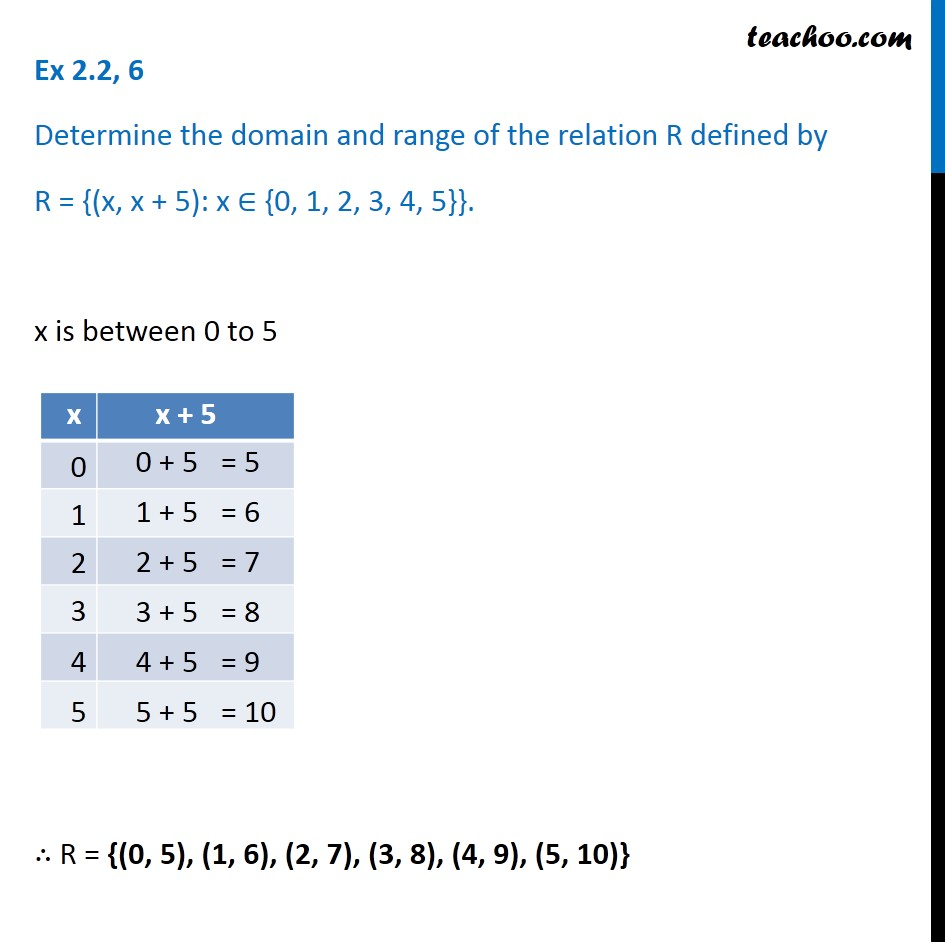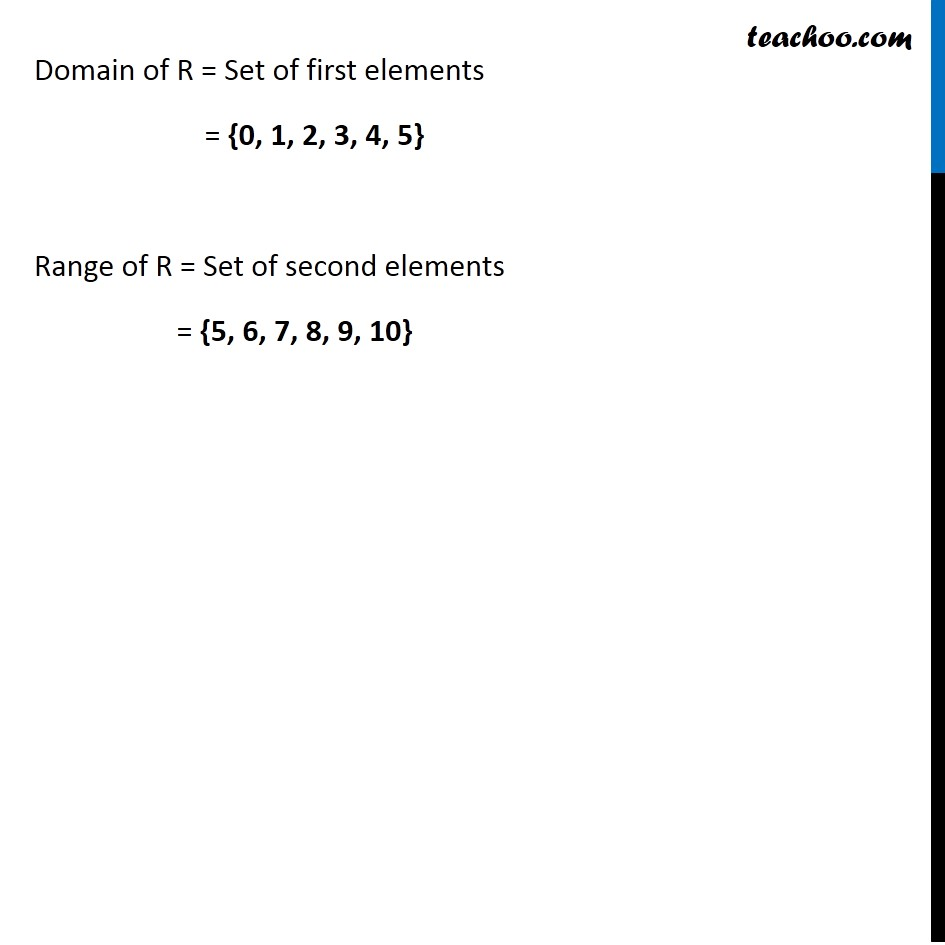1. Chapter 2 Class 11 Relations and Functions (Term 1)
2. Serial order wise
3. Ex 2.2

Transcript

Ex 2.2, 6 Determine the domain and range of the relation R defined by R = {(x, x + 5): x ∈ {0, 1, 2, 3, 4, 5}}. x is between 0 to 5 ∴ R = {(0, 5), (1, 6), (2, 7), (3, 8), (4, 9), (5, 10)} Domain of R = Set of first elements = {0, 1, 2, 3, 4, 5} Range of R = Set of second elements = {5, 6, 7, 8, 9, 10}

Ex 2.2

Chapter 2 Class 11 Relations and Functions (Term 1)
Serial order wise